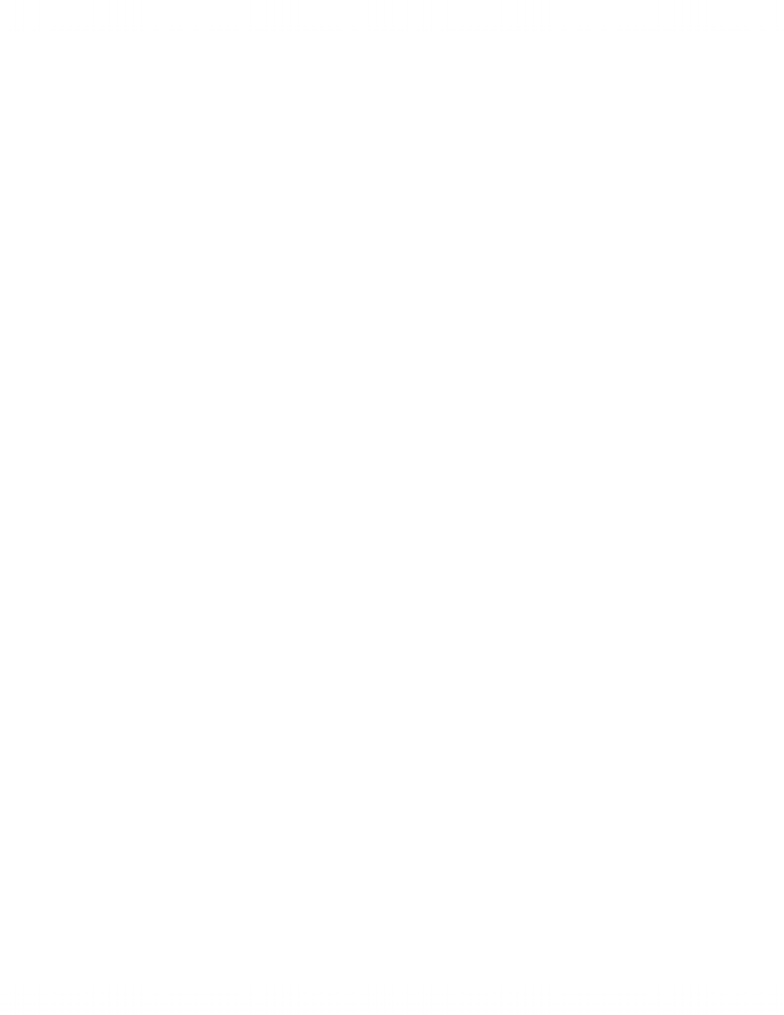# Economics 1021A/B Chapter Notes - Chapter 4: Demand Curve, Negative Number, Opportunity Cost

53 views6 pages
School
Western University
Department
Economics
Course
Economics 1021A/BMicroeconomics Chapter 4
Price Elasticity of Demand
different outcomes arise from differing degrees of responsiveness of the quantity demanded to
a change in price
the slope of a demand curve depends on the units in which we measure the price and quantity
units of measurement are unrelated, can’t compare demands for different goods
price elasticity of demand- a units-free measure of the responsiveness of the quantity
demanded of a good to a change in its price when all other influences on buying plans remain the
same
CALCULATING PRICE ELASTICITY OF DEMAND
price elasticity of demand = percentage change in quantity demanded/ percentage change in
price
to use this formula, need to know the quantities demanded at different prices
express the changes in price and quantity demanded as percentages of the average price and the
average quantity
AVERAGE PRICE AND QUANTITY
use average price and average quantity because it gives the most precise measurement of
elasticity- at the midpoint between the original price and the new price
get the same value for the elasticity regardless of whether the price falls or rises
PERCENTAGES AND PROPORTIONS
elasticity is the ratio of two percentage changes
when we divide one percentage change by another, the 100s cancel
a percentage change is a proportionate change multiplied by 100
the proportionate change in price is change in price/average price and the proportionate change
in quantity demanded is change in quantity/average quantity
A UNITS-FREE MEASURE
elasticity is a units-free measure because the percentage change in each variable is independent
of the units in which the variable is measure
MINUS SIGN AND ELASTICITY
positive change in price brings a negative change in the quantity demanded, the price elasticity
of demand is a negative number
the magnitude, or absolute value, of the price elasticity of demand that tells us how responsive
the quantity demanded is, ignore the minus sign
Inelastic and Elastic Demand
if the quantity demanded remains constant when the price changes, then the price elasticity of
demand is zero and the good is said to have a perfectly inelastic demand i.e. insulin
if the percentage change in the quantity demanded equals the percentage change in the price,
then the price elasticity is 1 and the good is said to have a unit elastic demand
the percentage change in the quantity demanded is more than the percentage change in the
price, price elasticity of demand is between 1 and infinity and the good is said to have an elastic
demand
if the quantity demanded changes by an infinitely large percentage is response to a tiny price
change, then the price elasticity of demand is infinity and the good is said to have a perfectly
elastic demand i.e. soft drink from two machines located side by side
Unlock document

This preview shows pages 1-2 of the document.
Unlock all 6 pages and 3 million more documents.the demand for a good that has a perfect substitute is perfectly elastic
the percentage change in the quantity demanded is less than the percentage change in the price,
price elasticity of demand is between zero and 1 and the good is said to have an inelastic demand
i.e. automobiles
Elasticity Along a Straight-Line Demand Curve
elasticity along a straight-line demand curve- a demand curve that has a constant slope
elasticity changes along a straight-line demand curve
at the midpoint of the curve, demand is unit elastic
above the midpoint, demand is elastic
below the midpoint, demand is inelastic
Total Revenue and Elasticity
total revenue from the sale of a good equals the price of the good multiplied by the quantity
sold
when a price changes, total revenue also changes
a rise in price does not always increase total revenue
if demand is elastic, a 1% price cut increases the quantity sold by more than 1% and total
revenue increases
if demand is inelastic, a 1% price cut increases the quantity sold by less than 1% and total
revenue decreases
if demand is unit elastic, a 1% price cut increases the quantity sold by 1% and total revenue
does not change
total revenue test- a method of estimating the price elasticity of demand by observing the
change in total revenue that results from a change in price, when all other influences on the
quantity sold remain the same
if a price cut increases total revenue, demand is elastic
if a price cut decreases total revenue, demand is inelastic
if a price cut leaves total revenue unchanged, demand is unit elastic
at unit elasticity, total revenue is at a maximum
if your demand is elastic, a 1% price cut increases the quantity you buy by more than 1% and
your expenditure on the item increases
if your demand is inelastic, a 1% price cut increases the quantity you buy by less than 1% and
your expenditure on the item decreases
if your demand is unit elastic, a 1% price cut increases the quantity you buy by 1% and your
expenditure on the item does not change
The Factors that Influence the Elasticity of Demand
elasticity of demand for a good depends on the closeness of substitutes, proportion of income
spent on the good and time elapsed since a price change
CLOSENESS OF SUBSTITUTES
Unlock document

This preview shows pages 1-2 of the document.
Unlock all 6 pages and 3 million more documents.